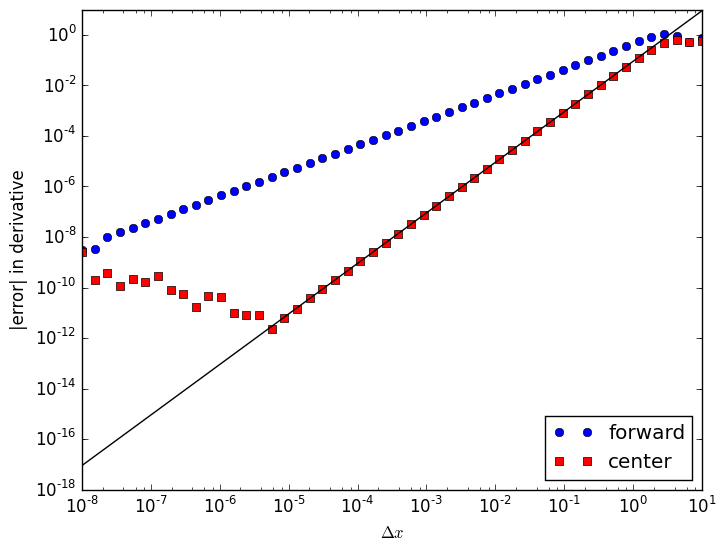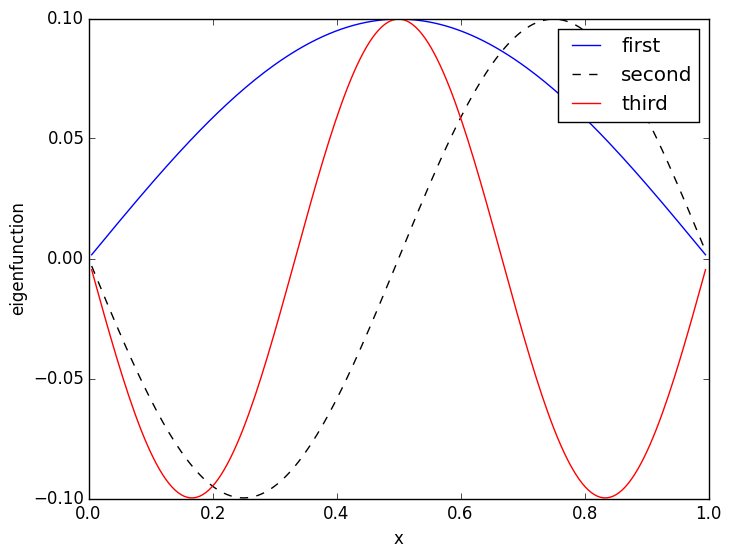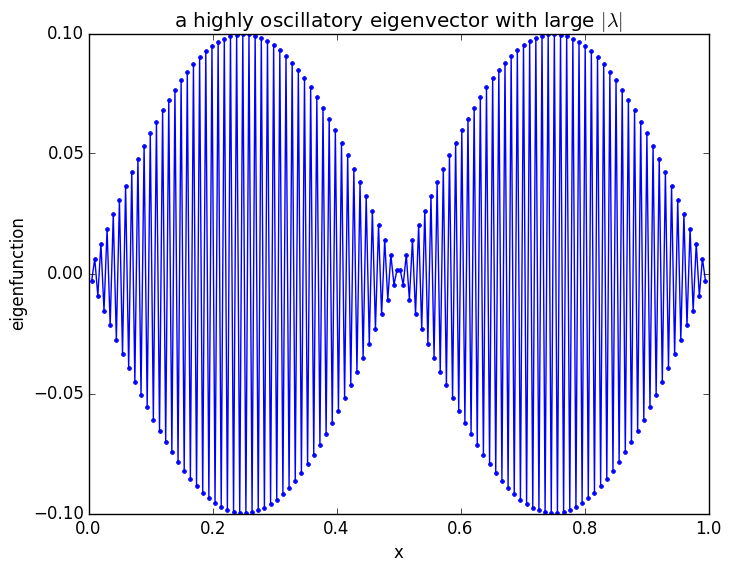Finite differences¶

Similar to the handout from class, let's compute forward- and center-difference approximations for the first derivative $f'(x)$ of the function $f(x) = \sin(x)$. In particular, we'll compute $f'(1)$ compared to the exact result $\cos(1)$ as a function of the step size $\Delta x$.

In :
x = 1
Δx = logspace(-8, 1, 50)                # 50 logarithmically spaced points from 0.00000001 to 10 (type Δx via \Delta<TAB>x)
f = (sin(x+Δx) - sin(x)) ./ Δx          # forward difference ... note that ./ means element-wise division
c = (sin(x+Δx) - sin(x-Δx)) ./ (2Δx)    # center difference

using PyPlot
loglog(Δx, abs(cos(x) - f), "bo")
loglog(Δx, abs(cos(x) - c), "rs")
loglog(Δx, Δx.^2 * cos(x)/6, "k-")      # plot center-difference error estimate from Taylor expansion
xlabel(L"$\Delta x$")
ylabel("|error| in derivative")
legend(["forward", "center"], loc="lower right")Out:
PyObject <matplotlib.legend.Legend object at 0x314b76d10>

Finite-difference Laplacian¶

As in class, we apply the center-difference approximation twice to approximate $\hat{A} = \frac{\partial^2}{\partial x^2}$ by a matrix $A$ on $N$ equally spaced points on $x \in [0,L]$, for functions $u(x)$ with Dirichlet boundary conditions $u(0) = u(L) = 0$.

(The diagm(v, n) command in Julia takes a vector v and makes a square matrix with v on the n-th diagonal.)

In :
N = 200
L = 1
Δx = L / (N+1)
A = (-2*diagm(ones(N)) + diagm(ones(N-1),1) + diagm(ones(N-1),-1)) / (Δx)^2
Out:
200x200 Array{Float64,2}:
-80802.0   40401.0       0.0       0.0  …       0.0       0.0       0.0
40401.0  -80802.0   40401.0       0.0          0.0       0.0       0.0
0.0   40401.0  -80802.0   40401.0          0.0       0.0       0.0
0.0       0.0   40401.0  -80802.0          0.0       0.0       0.0
0.0       0.0       0.0   40401.0          0.0       0.0       0.0
0.0       0.0       0.0       0.0  …       0.0       0.0       0.0
0.0       0.0       0.0       0.0          0.0       0.0       0.0
0.0       0.0       0.0       0.0          0.0       0.0       0.0
0.0       0.0       0.0       0.0          0.0       0.0       0.0
0.0       0.0       0.0       0.0          0.0       0.0       0.0
0.0       0.0       0.0       0.0  …       0.0       0.0       0.0
0.0       0.0       0.0       0.0          0.0       0.0       0.0
0.0       0.0       0.0       0.0          0.0       0.0       0.0
⋮                                  ⋱
0.0       0.0       0.0       0.0          0.0       0.0       0.0
0.0       0.0       0.0       0.0          0.0       0.0       0.0
0.0       0.0       0.0       0.0  …       0.0       0.0       0.0
0.0       0.0       0.0       0.0          0.0       0.0       0.0
0.0       0.0       0.0       0.0          0.0       0.0       0.0
0.0       0.0       0.0       0.0          0.0       0.0       0.0
0.0       0.0       0.0       0.0          0.0       0.0       0.0
0.0       0.0       0.0       0.0  …       0.0       0.0       0.0
0.0       0.0       0.0       0.0      40401.0       0.0       0.0
0.0       0.0       0.0       0.0     -80802.0   40401.0       0.0
0.0       0.0       0.0       0.0      40401.0  -80802.0   40401.0
0.0       0.0       0.0       0.0          0.0   40401.0  -80802.0

We'll use the eig command to compute the eigenvalues λ (typed as \lambda followed by tab) and the eigenvectors X (X is a matrix whose columns are the eigenvectors.)

In :
λ, X = eig(A)
Out:
([-1.61594e5,-1.61565e5,-1.61515e5,-1.61446e5,-1.61357e5,-161249.0,-1.61121e5,-1.60973e5,-1.60806e5,-160619.0  …  -984.953,-798.121,-630.832,-483.128,-355.045,-246.615,-157.862,-88.8102,-39.4752,-9.8694],
200x200 Array{Float64,2}:
0.00155903  -0.00311767   0.00467555  …  -0.00311767  0.00155903
-0.00311767   0.00623229  -0.00934083     -0.00623229  0.00311767
0.00467555  -0.00934083   0.0139856      -0.00934083  0.00467555
-0.00623229   0.0124402   -0.0185996      -0.0124402   0.00623229
0.00778751  -0.0155275    0.0231727      -0.0155275   0.00778751
-0.00934083   0.0185996   -0.0276948   …  -0.0185996   0.00934083
0.0108919   -0.0216535    0.0321561      -0.0216535   0.0108919
-0.0124402    0.0246862   -0.0365468      -0.0246862   0.0124402
0.0139856   -0.0276948    0.040857       -0.0276948   0.0139856
-0.0155275    0.0306764   -0.0450775      -0.0306764   0.0155275
0.0170656   -0.033628     0.0491988   …  -0.033628    0.0170656
-0.0185996    0.0365468   -0.0532121      -0.0365468   0.0185996
0.020129    -0.0394298    0.0571083      -0.0394298   0.020129
⋮                                     ⋱
0.0185996    0.0365468    0.0532121       0.0365468   0.0185996
-0.0170656   -0.033628    -0.0491988       0.033628    0.0170656
0.0155275    0.0306764    0.0450775   …   0.0306764   0.0155275
-0.0139856   -0.0276948   -0.040857        0.0276948   0.0139856
0.0124402    0.0246862    0.0365468       0.0246862   0.0124402
-0.0108919   -0.0216535   -0.0321561       0.0216535   0.0108919
0.00934083   0.0185996    0.0276948       0.0185996   0.00934083
-0.00778751  -0.0155275   -0.0231727   …   0.0155275   0.00778751
0.00623229   0.0124402    0.0185996       0.0124402   0.00623229
-0.00467555  -0.00934083  -0.0139856       0.00934083  0.00467555
0.00311767   0.00623229   0.00934083      0.00623229  0.00311767
-0.00155903  -0.00311767  -0.00467555      0.00311767  0.00155903)

The eigenvalues are all negative and are sorted in increasing order, so that the eigenvalues of smallest magnitude are last. flipdim(λ,1) reverses the order, so that the smallest-magnitude eigenvalues are first. We then take the first five of these eigenvalues (1:5 denotes the indices 1,2,3,4,5) and compare them to the exact eigenvalues $-(n\pi/L)^2$ of $\partial^2/\partial x^2$:

In :
(flipdim(λ,1)[1:5] - -((1:5) * π / L).^2) ./ (flipdim(λ,1)[1:5])
Out:
5-element Array{Float64,1}:
-2.03578e-5
-8.14343e-5
-0.000183238
-0.000325785
-0.000509095

If we go back and double $N$ (halving $\Delta x$), we'll find that the error becomes smaller by roughly a factor of 4, just as we would expect from the second-order convergence of the center-difference approximation we used.

Notice that the fractional error (the difference divided by $\lambda$) in the eigenvalue increases with increasing $n$. The reason is that larger-$n$ eigenfunctions are more oscillatory, and the same $\Delta x$ gives a worse approximation for the derivative if the function is oscillating faster compared to the grid.

Now, let's plot a few of the eigenfunctions corresponding to the last few eigenvalues, i.e. the smallest-$|\lambda|$ eigenvalues:

In :
x = [1:N]*Δx
plot(x, X[:,end], "b-")
plot(x, X[:,end-1], "k--")
plot(x, X[:,end-2], "r-")
xlabel("x")
ylabel("eigenfunction")
legend(["first", "second", "third"])Out:
PyObject <matplotlib.legend.Legend object at 0x3154e2ed0>

As we might have hoped, these are very good approximations for the exact eigenfunctions $\sin(n\pi x/L)$ ($n=1,2,3$) of the second-derivative operator.

In contrast, if we look at one of the largest-$|\lambda|$ eigenvectors, we get something highly oscillatory that doesn't much resemble the corresponding eigenfunction of $\partial^2/\partial x^2$. This eigenfunction oscillates in sign from one grid point to the next, and the finite-difference approximation is nothing like a derivative:

In :
plot(x, X[:,2], "b.-")
xlabel("x")
ylabel("eigenfunction")
title(L"a highly oscillatory eigenvector with large $|\lambda|$")Out:
PyObject <matplotlib.text.Text object at 0x31554b950>
In [ ]: